Question

Let B = {bį, b2} and C = {C1,C2} be bases for R², where b, -6--0--0--01...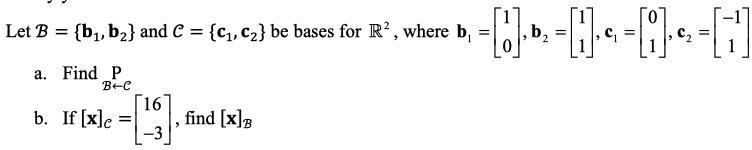Let B = {bį, b2} and C = {C1,C2} be bases for R², where b, -6--0--0--01 1 a. Find P BEC [16 b. If [x]c = -3 de= , find [x]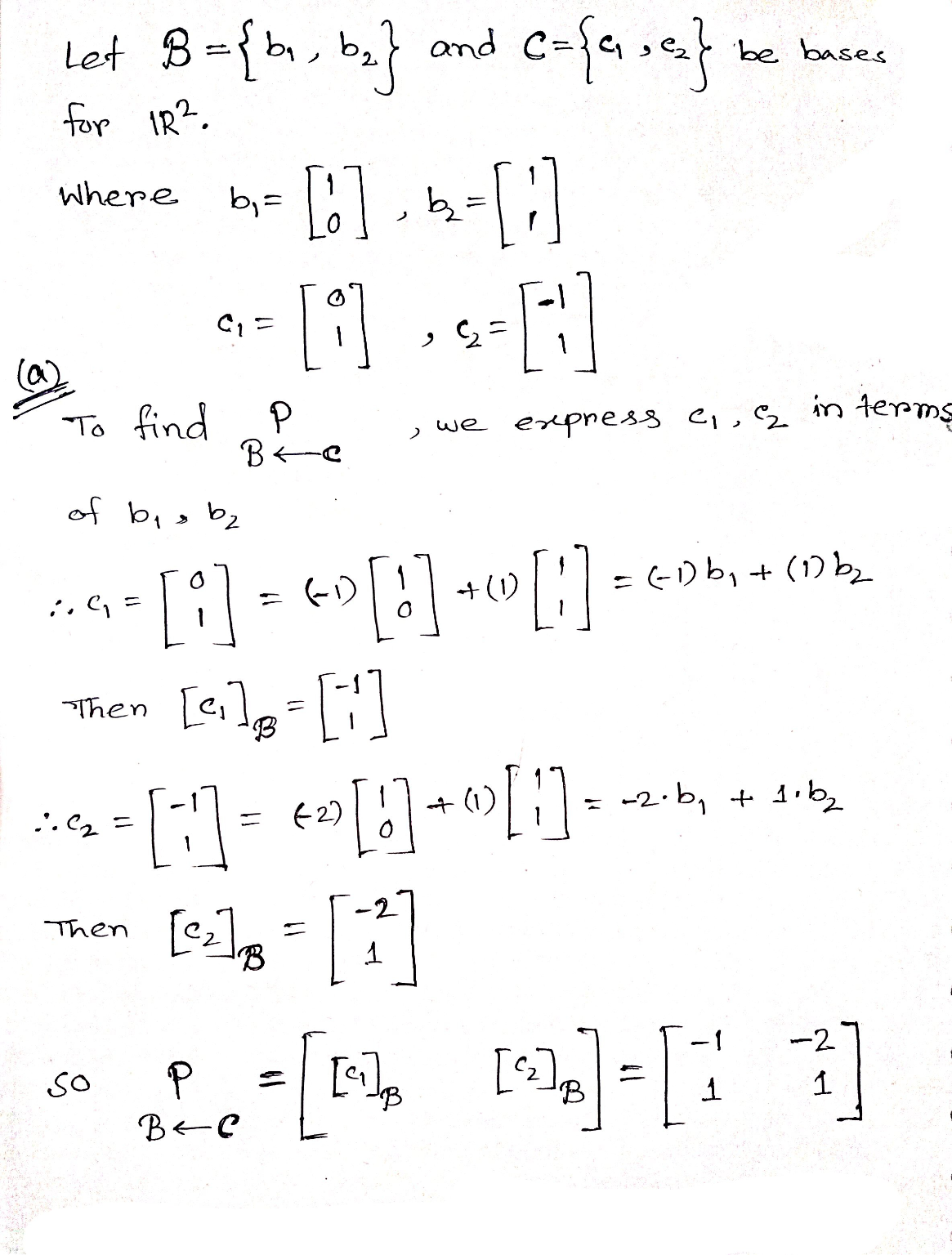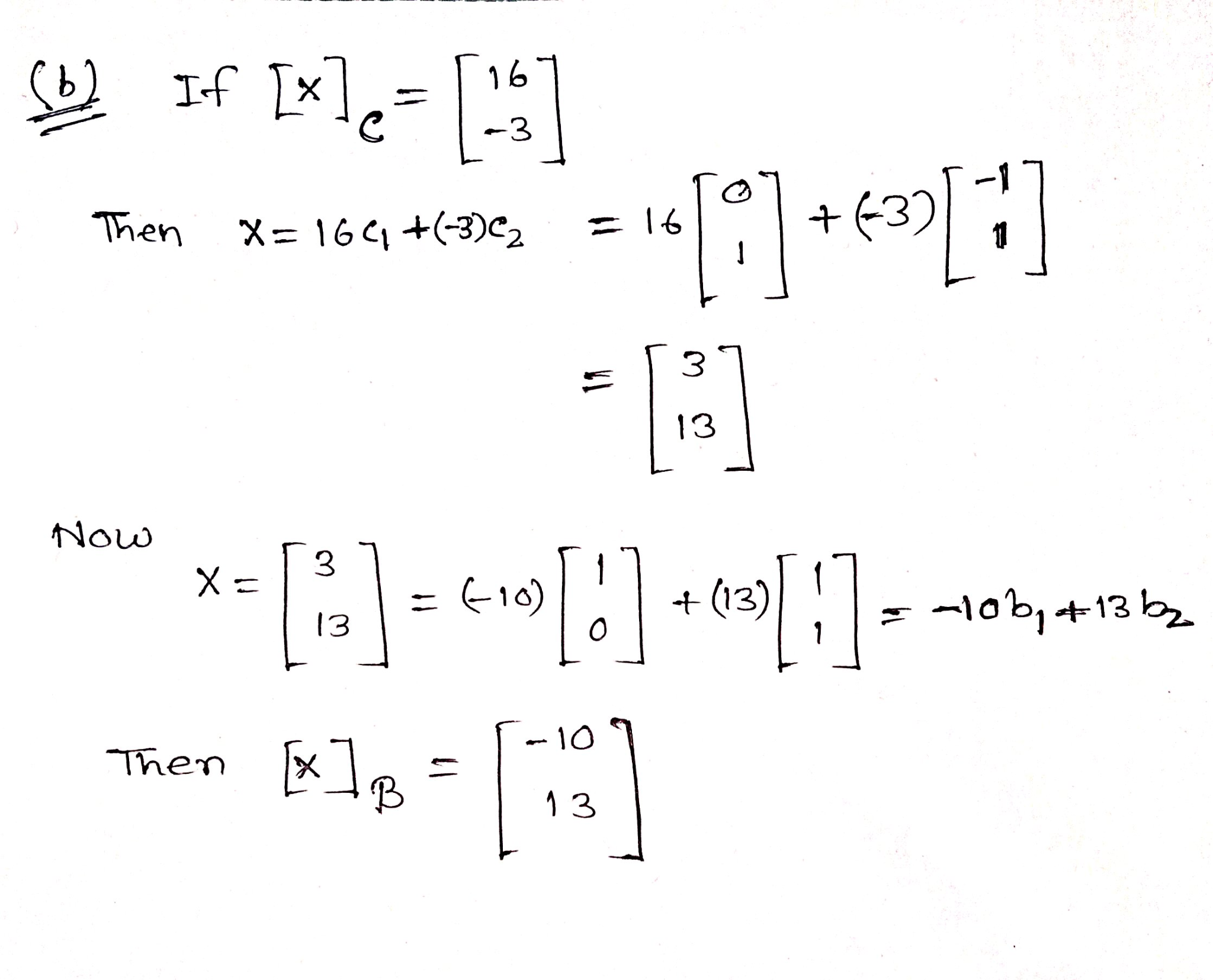Earn Coins

Coins can be redeemed for fabulous gifts.

Similar Homework Help Questions
• Question 6. (15 pts) Let B = {bı, b2} and C = {ci, c2} be bases...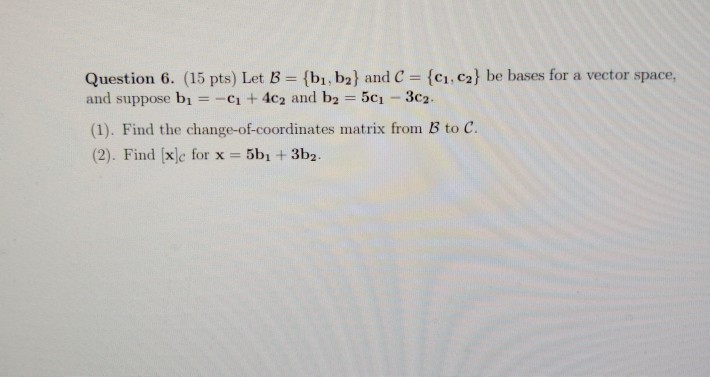Question 6. (15 pts) Let B = {bı, b2} and C = {ci, c2} be bases for a vector space, and suppose bı = - + 4c2 and b2 = 501 - 3c2. (1). Find the change-of-coordinates matrix from B to C. (2). Find [x]c for x = 5bı + 3b2.

• Assume that the transition matrix from basis B = {b1, b2, b3} to basis C = {c1, c2, c3} is PC,B = 1/2*[ 0 -1 1 ; -1 1 1 ; 1 0 0 ]. (a) If u = b1 + b2 + 2b3, find [u]C. (b) Calculate PB,C. (c) Suppose...

Assume that the transition matrix from basis B = {b1, b2, b3} to basis C = {c1, c2, c3} is PC,B = 1/2*[ 0 -1 1 ; -1 1 1 ; 1 0 0 ]. (a) If u = b1 + b2 + 2b3, find [u]C. (b) Calculate PB,C. (c) Suppose that c1 = (1, 2, 3), c2 = (1, 2, 0), c3 = (1, 0, 0) and let S be the standard basis for R 3 . (i) Find...

• Let B = {b1,b2} and C= {(1,62} be bases for R2. Find the change-of-coordinates matrix from...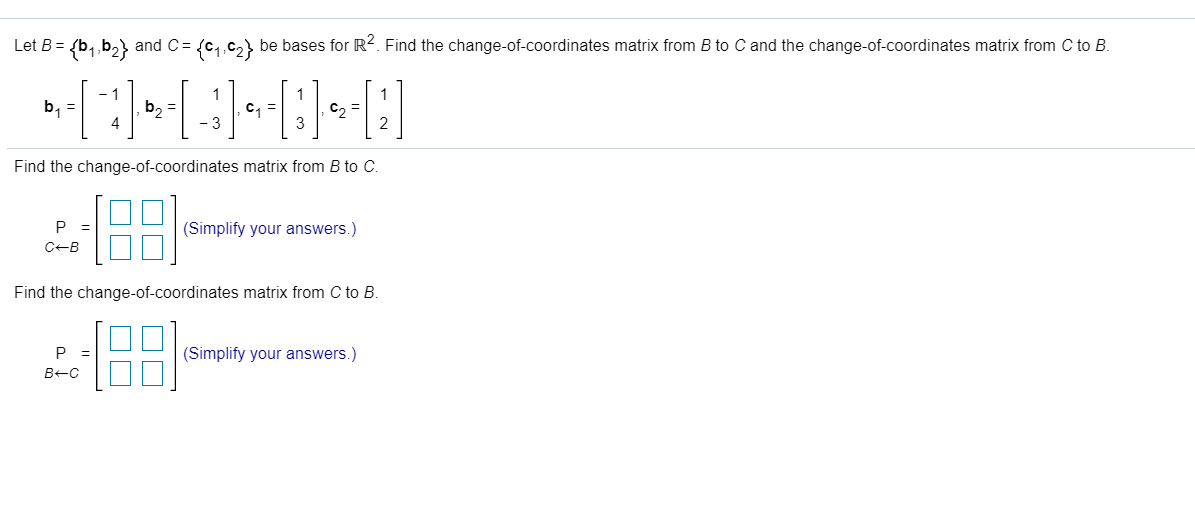Let B = {b1,b2} and C= {(1,62} be bases for R2. Find the change-of-coordinates matrix from B to C and the change-of-coordinates matrix from C to B. - 1 b = b2 = C1 = C = 4 -3 Find the change-of-coordinates matrix from B to C. P = CB (Simplify your answers.) Find the change-of-coordinates matrix from C to B. P B-C [8: (Simplify your answers.)

• 1. Let B1 = {1, æ, } and B2 = {x - 1,22 - 1 -...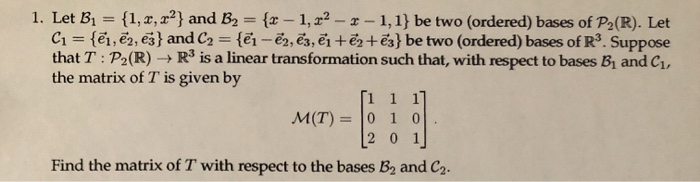1. Let B1 = {1, æ, } and B2 = {x - 1,22 - 1 - 1,1} be two (ordered) bases of P2(R). Let C1 = {ēm, ēm, ēj} and C2 = {@i - ēm, ēz, ?i + ?2+ēz} be two (ordered) bases of R3. Suppose the matrix of T is given by [1 1 1] [2 0 1 Find the matrix of T with respect to the bases B, and C.

• Let R be the region shown above bounded by the curve C = C1[C2. C1 is a semicircle with center at...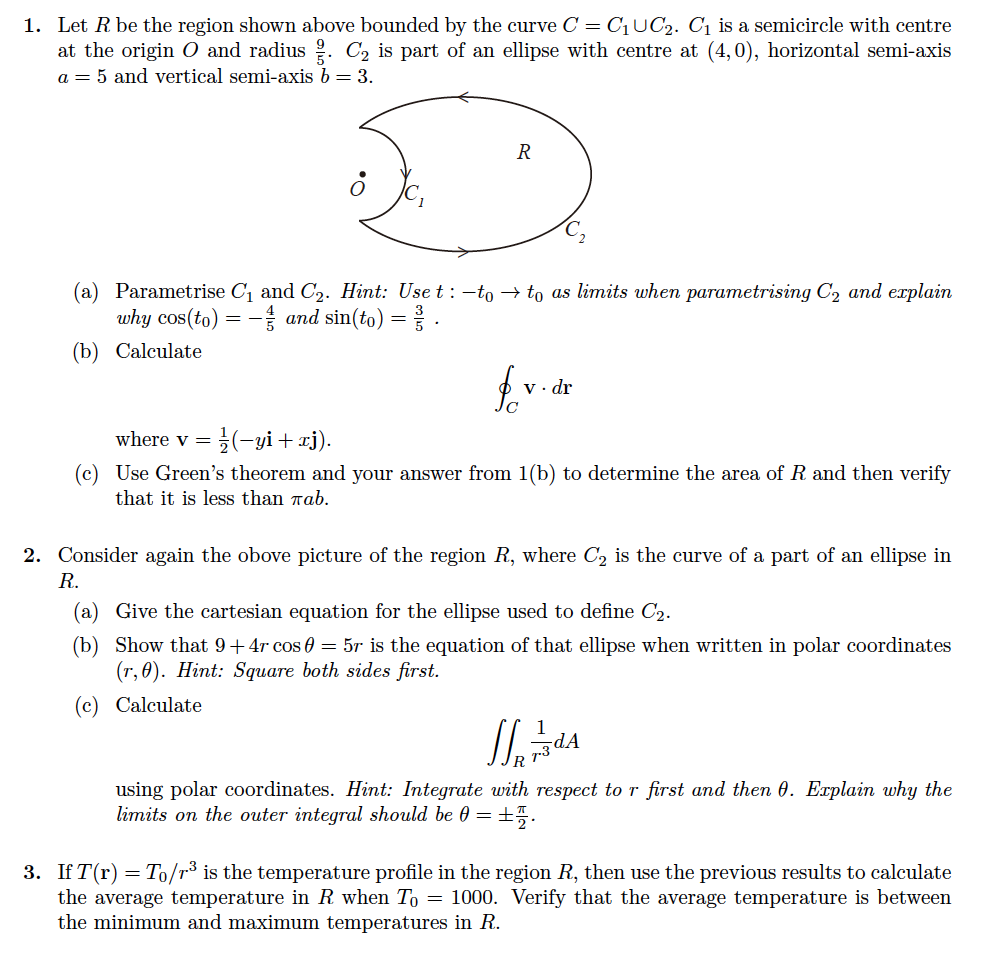Let R be the region shown above bounded by the curve C = C1[C2. C1 is a semicircle with center at the origin O and radius 9 5 . C2 is part of an ellipse with center at (4; 0), horizontal semi-axis a = 5 and vertical semi-axis b = 3. Thanks a lot for your help:) 1. Let R be the region shown above bounded by the curve C - C1 UC2. C1 is a semicircle with centre at...

• Exercise 4 Leta(c)-c1/2 and let c2 > cı > 0 be given. Let: π1c1+12c2. where π2...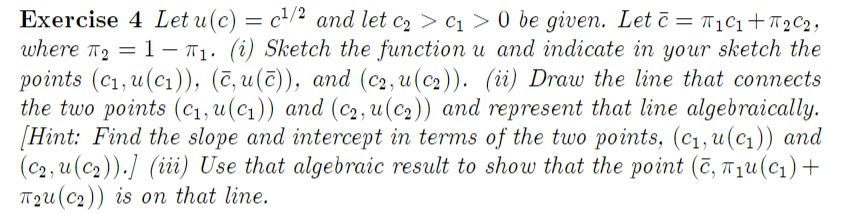Exercise 4 Leta(c)-c1/2 and let c2 > cı > 0 be given. Let: π1c1+12c2. where π2 = 1-T1. (i) Sketch the function u and indicate in your sketch the points (C1, u(a), (c, u(c)), and (c2,u(c2)). (ii) Draw the line that connects the two points (ci, u(cı)) and (c2, u(c2)) and represent that line algebraically. Hint: Find the slope and intercept in terms of the two points, (c1, u(c) and (c,,u (сг)).] (iii) Use that algebraic result to show that...

• 9. Let S = {C1, C2, C3, es} be the standard basis for R, and let...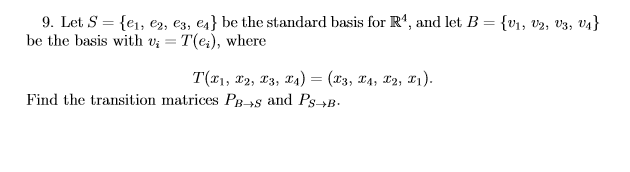9. Let S = {C1, C2, C3, es} be the standard basis for R, and let B = {V1, 02, 03, 04} be the basis with vi = T(e), where T(21, 12, 13, 14) = (x3, 14, 20, 21). Find the transition matrices PB +and Ps+B.

• (b) Let f 0, 1-R be a C2 function and let g, h: [0, 00)-R be C1. Consider the initial-boundary value problem kwr w(...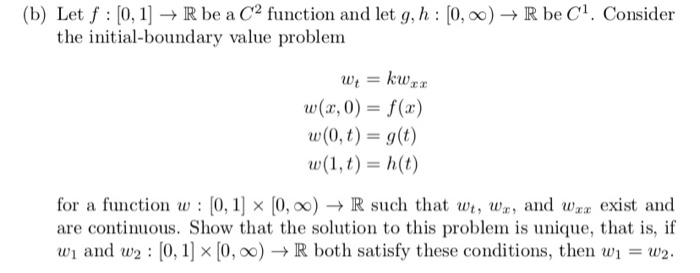(b) Let f 0, 1-R be a C2 function and let g, h: [0, 00)-R be C1. Consider the initial-boundary value problem kwr w(r, 0) f(a) w(0, t) g(t) w(1, t) h(t) for a function w: [0,1 x [0, 0)- R such that w, wn, and wa exist and are continuous. Show that the solution to this problem is unique, that is, if w1 and w2 [0, 1] x [0, 00)- R both satisfy these conditions, then w1 = w2....

• 1) for R2 Given the vectors b1,b2, C1, and cz. B = {b1,b2} is a basis...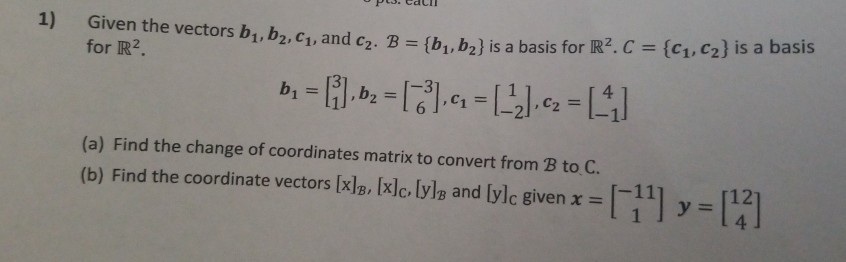1) for R2 Given the vectors b1,b2, C1, and cz. B = {b1,b2} is a basis for R2C = {C1,C2} is a basis b = [i.bz = ,4 = (-2) c2 =  (a) Find the change of coordinates matrix to convert from B to C. (b) Find the coordinate vectors [x]B, [x]c, lyle and [ylc given x =  y = 

• C1= 5 C2= 6 C3= 10 GCD --> Greater Common Divisor B1 a. Let x :=...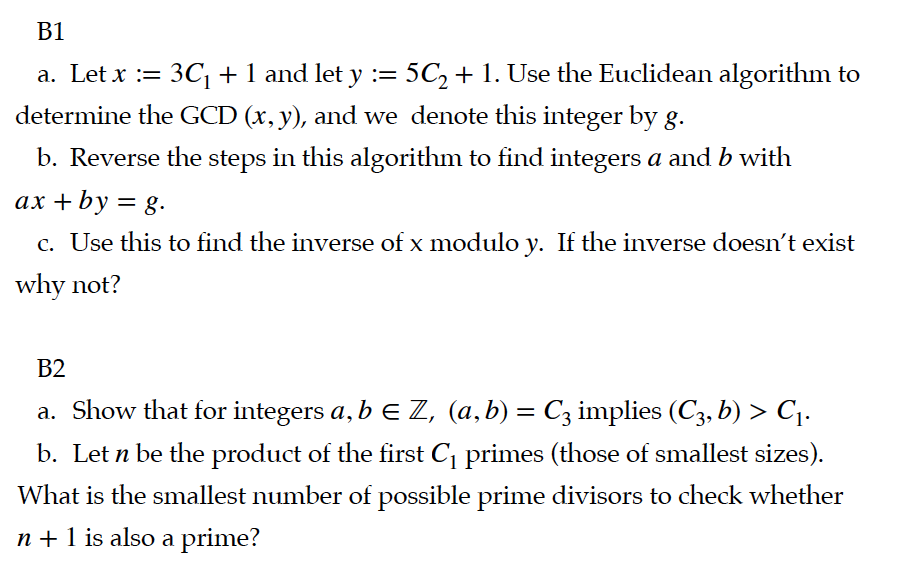C1= 5 C2= 6 C3= 10 GCD --> Greater Common Divisor B1 a. Let x := 3C1 + 1 and let y := 5C2 + 1. Use the Euclidean algorithm to determine the GCD (x, y), and we denote this integer by g. b. Reverse the steps in this algorithm to find integers a and b with ax + by = g. c. Use this to find the inverse of x modulo y. If the inverse doesn't exist why not?...